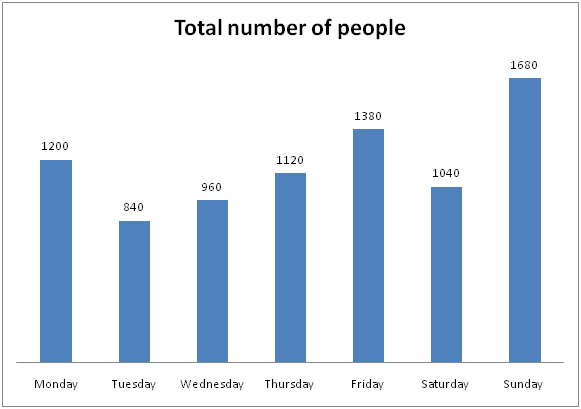# CWC/FCI Prelims 2019 – Quantitative Aptitude Questions (Day-14)

Dear Readers, Exam Race for the Year 2019 has already started, To enrich your preparation here we are providing new series of Practice Questions on Quantitative Aptitude – Section for CWC/FCI Exam. Aspirants, practice these questions on a regular basis to improve your score in aptitude section. Start your effective preparation from the right beginning to get success in upcoming CWC/FCI Exam.

[WpProQuiz 5532]

Directions (Q. 1 – 5): What value should come in place of question mark (?) in the following questions?

1) 34 % of 4200 – 510 ÷ ? + 72 % of 400 = 1713

a) 280

b) 320

c) 170

d) 120

e) None of these

2) (5/8) of (16/9) of (18/5) of 65 % of 800 =?

a) 2080

b) 2260

c) 1820

d) 1640

e) None of these

3) (11340 ÷ 9) + 85 % of 1600 – (5/7) of 2100 =? – 112

a) 1364

b) 1057

c) 1125

d) 1241

e) None of these

4) 5 (¾) + 6 (½) – 3 (3/8) + 4 (5/8) = ? + 1 (½)

a) 15 (¾)

b) 12

c) 16

d) 17 (½)

e) None of these

5) 30 % of 5400 + 68 % of 4100 =? – 2168

a) 6576

b) 7154

c) 6243

d) 5812

e) None of these

Directions (Q. 6 – 10): Study the following information carefully and answer the given questions:

The following bar graph shows the total number of people (Male and Female) went to a theatre in 7 different days of a week.6) If the ratio between the total number of male to that of female went to the theatre on Monday and Wednesday is 8 : 7 and 5 : 7 respectively, then the total number of female went to the theatre on Monday is what percentage of total number of male went to the theatre on Wednesday?

a) 120 %

b) 105 %

c) 140 %

d) 155 %

e) None of these

7) Find the average number of people went for the theatre in the week except Sunday?

a) 1120

b) 1350

c) 1240

d) 1090

e) None of these

8) Find the ratio of the total number of people went for the theatre on Monday, Wednesday and Friday together to that of total number of people went for the theatre on Tuesday, Thursday and Sunday together?

a) 52: 59

b) 177: 182

c) 28: 35

d) 7: 17

e) None of these

9) If 45 % and 30 % of people went for a theatre on Thursday and Friday is booked AC tickets respectively, then find the total number of people booked AC tickets on Thursday and Friday together?

a) 852

b) 934

c) 918

d) 976

e) None of these

10) Total number of people went for a theatre on Sunday and Monday together is approximately what percentage more/less than the total number of people went for a theatre on Tuesday and Thursday together?

a) 47 % more

b) 38 % less

c) 59 % less

d) 38 % more

e) 47 % less

Direction (1-5) :

34 % of 4200 – 510 ÷ x + 72 % of 400 = 1713

(34/100)*4200 – (510/x) + (72/100)*400 = 1713

1428 + 288 – 1713 = (510/x)

3 = (510/x)

x = 510/3 = 170

(5/8)*(16/9)*(18/5)*(65/100)*800 = x

X = 2080

(11340/9) + (85/100)*1600 – (5/7)*2100 = x – 121

1260 + 1360 – 1500 + 121 = x

X = 1241

5 (¾) + 6 (½) – 3 (3/8) + 4 (5/8) – 1 (½) = x

X = (5 + 6 – 3 + 4 – 1) + (3/4 + ½ – 3/8 + 5/8 – 1/2)

X = 11 + (6 + 4 – 3 + 5 – 4)/8 = 11 + (8/8) = 12

30 % of 5400 + 68 % of 4100 = x – 2168

(30/100)*5400 + (68/100)*4100 + 2168 = x

X = 1620 + 2788 + 2168 = 6576

Direction (6-10) :

The total number of female went to the theatre on Monday

= > 1200*(7/15) = 560

The total number of male went to the theatre on Wednesday

= > 960*(5/12) = 400

Required % = (560/400)*100 = 140 %

The total number of people went for the theatre in the week except Sunday

= > 1200 + 840 + 960 + 1120 + 1380 + 1040 = 6540

Required average = 6540/6 = 1090

The total number of people went for the theatre on Monday, Wednesday and Friday together

= > 1200 + 960 + 1380 = 3540

The total number of people went for the theatre on Tuesday, Thursday and Sunday together

= > 840 + 1120 + 1680 = 3640

Required ratio = 3540: 3640 = 177: 182

The total number of people booked AC tickets on Thursday and Friday together

= > 1120*(45/100) + 1380*(30/100)

= > 504 + 414 = 918

Total number of people went for a theatre on Sunday and Monday together

= > 1680 + 1200 = 2880

Total number of people went for a theatre on Tuesday and Thursday together

= > 840 + 1120 = 1960

Required % = [(2880 – 1960)/1960]*100 = 47 % more